# Engineering Mechanics - PKRB: Impulse and Momentum

## Why Engineering Mechanics PKRB: Impulse and Momentum?

In this section you can learn and practice Engineering Mechanics Questions based on "PKRB: Impulse and Momentum" and improve your skills in order to face the interview, competitive examination and various entrance test (CAT, GATE, GRE, MAT, Bank Exam, Railway Exam etc.) with full confidence.

## Where can I get Engineering Mechanics PKRB: Impulse and Momentum questions and answers with explanation?

IndiaBIX provides you lots of fully solved Engineering Mechanics (PKRB: Impulse and Momentum) questions and answers with Explanation. Solved examples with detailed answer description, explanation are given and it would be easy to understand. All students, freshers can download Engineering Mechanics PKRB: Impulse and Momentum quiz questions with answers as PDF files and eBooks.

## Where can I get Engineering Mechanics PKRB: Impulse and Momentum Interview Questions and Answers (objective type, multiple choice)?

Here you can find objective type Engineering Mechanics PKRB: Impulse and Momentum questions and answers for interview and entrance examination. Multiple choice and true or false type questions are also provided.

## How to solve Engineering Mechanics PKRB: Impulse and Momentum problems?

You can easily solve all kind of Engineering Mechanics questions based on PKRB: Impulse and Momentum by practicing the objective type exercises given below, also get shortcut methods to solve Engineering Mechanics PKRB: Impulse and Momentum problems.

### Exercise :: PKRB: Impulse and Momentum - General Questions

• PKRB: Impulse and Momentum - General Questions
1.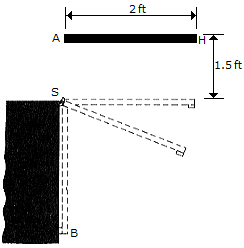The uniform rod AB has a weight of 3 lb and is released from rest without rotating from the position shown. As it falls, the end A strikes a hook S, which provides a permanent connection. Determine the speed at which the other end B strikes the wall at C.

 A. vB4 = 14.74 ft/s B. vB4 = 20.3 ft/s C. vB4 = 22.0 ft/s D. vB4 = 12.69 ft/s

Explanation:

No answer description available for this question. Let us discuss.

2.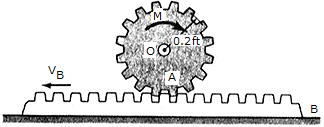Gear A has a weight of 1.5 lb, a radius of 0.2 ft, and a radius of gyration of ko = 0.13ft. The coefficient of friction between the gear rack B and the horizontal surface is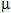= 0.3. If the rack has a weight of 0.8 lb and is initially sliding to the left with a velocity of (vB)2 = 8 ft/s to the left. Neglect friction between the rack and the gear and assume that the gear exerts only a horizontal force on the rack.

 A. M = 0.01425 lb-ft B. M = 0.00630 lb-ft C. M = 0.0622 lb-ft D. M = 0.0560 lb-ft

Explanation:

No answer description available for this question. Let us discuss.

3.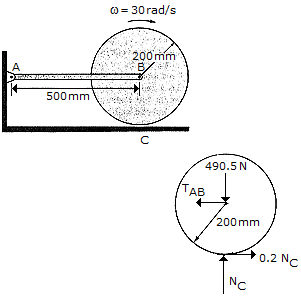The 50-kg cylinder has an angular velocity of 30 rad/s when it is brought into contact with the horizontal surface at C. If the coefficient of friction isc = 0.2, determine how long it takes for the cylinder to stop spinning. What force is developed at the pin A during this time? The axis of the cylinder is connected to two symmetrical links. (Only AB is shown.) For the computation, neglect the weight of the links.

 A. t = 1.529 s, A = 0 B. t = 3.06 s, A = 0 C. t = 1.529 s, A = 49.1 N D. t = 3.06 s, A = 49.1 N

Explanation:

No answer description available for this question. Let us discuss.

4.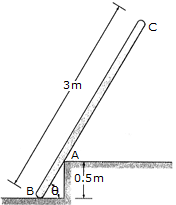The uniform pole has a mass of 15 kg and falls from rest when= 90° until it strikes the edge at A,= 60°. If the pole then begins to pivot about this point after contact, determine the pole's angular velocity just after the impact. Assume that the pole does not slip at B as it falls until it strikes A.

 A.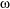3 = 1.146 rad/s B.3 = 0.537 rad/s C.3 = 2.15 rad/s D.3 = 1.528 rad/s

Explanation:

No answer description available for this question. Let us discuss.

5.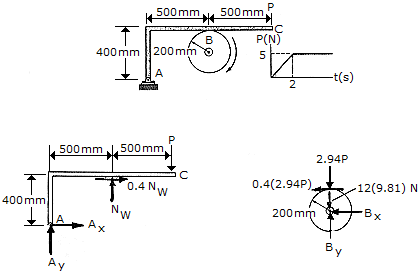The 12-kg disk has an angular velocity of= 20 rad/s. If the brake ABC is applied such that the magnitude of force P varies with time as shown, determine the time needed to stop the disk. The coefficient of friction at B is= 0.4.

 A. t = 13.00 s B. t = 7.00 s C. t = 8.92 s D. t = 5.08 s